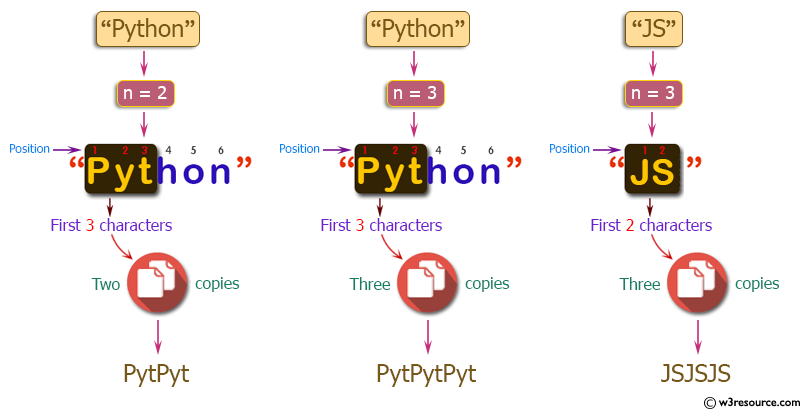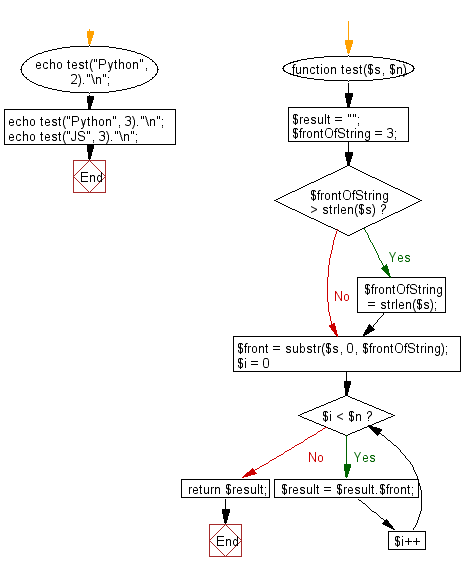﻿ PHP Exercises: Create a new string which is n copies of the the first 3 characters of a given string - w3resource# PHP Exercises: Create a new string which is n copies of the the first 3 characters of a given string

## PHP Basic Algorithm: Exercise-26 with Solution

Write a PHP program to create a new string which is n (non-negative integer) copies of the the first 3 characters of a given string. If the length of the given string is less than 3 then return n copies of the string.

Sample Solution:

PHP Code :

``````<?php
function test(\$s, \$n)
{
\$result = "";
\$frontOfString = 3;

if (\$frontOfString > strlen(\$s))
\$frontOfString = strlen(\$s);

\$front = substr(\$s, 0, \$frontOfString);

for (\$i = 0; \$i < \$n; \$i++)
{
\$result = \$result.\$front;
}
return \$result;
}

echo test("Python", 2)."\n";
echo test("Python", 3)."\n";
echo test("JS", 3)."\n";
``````

Sample Output:

```PytPyt
PytPytPyt
JSJSJS
```

Pictorial Presentation:Flowchart:PHP Code Editor:

What is the difficulty level of this exercise?

﻿

## PHP: Tips of the Day

\$_REQUEST: This SuperGlobal Variable is used to collect data submitted by a HTML Form

Example:

This code save in a php file and run in the browser.

```<!DOCTYPE html>
<html>
<body>

<form method="post" action="<?php echo \$_SERVER['PHP_SELF'];?>">
NAME: <input type="text" name="user">
<button type="submit">SUBMIT</button>
</form>
<?php
if (\$_SERVER["REQUEST_METHOD"] == "POST") {
\$name = htmlspecialchars(\$_REQUEST['user']);
if(empty(\$name)){
echo "Name is empty";
} else {
echo \$name;
}
}
?>
</body>
</html>
```

Output:

```Owen
```